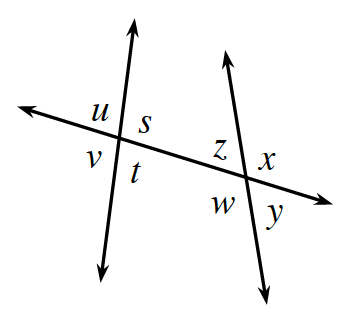### Home > CCG > Chapter 6 > Lesson 6.1.1 > Problem6-4

6-4.Use the diagram at right to answer the following questions.

Review the Math Notes box in Lesson 2.1.4.

1. What type of angle pair is $∠z$ and $∠t$? That is, what is their geometric relationship?

2. What type of angle pair do $∠s$ and $∠v$ form?

3. Name all pairs of corresponding angles in the diagram. Hint: There are four different pairs.

$∠u$ and $∠z$, $∠s$ and $∠x$, $∠v$ and $∠w$, ∠t and $∠y$